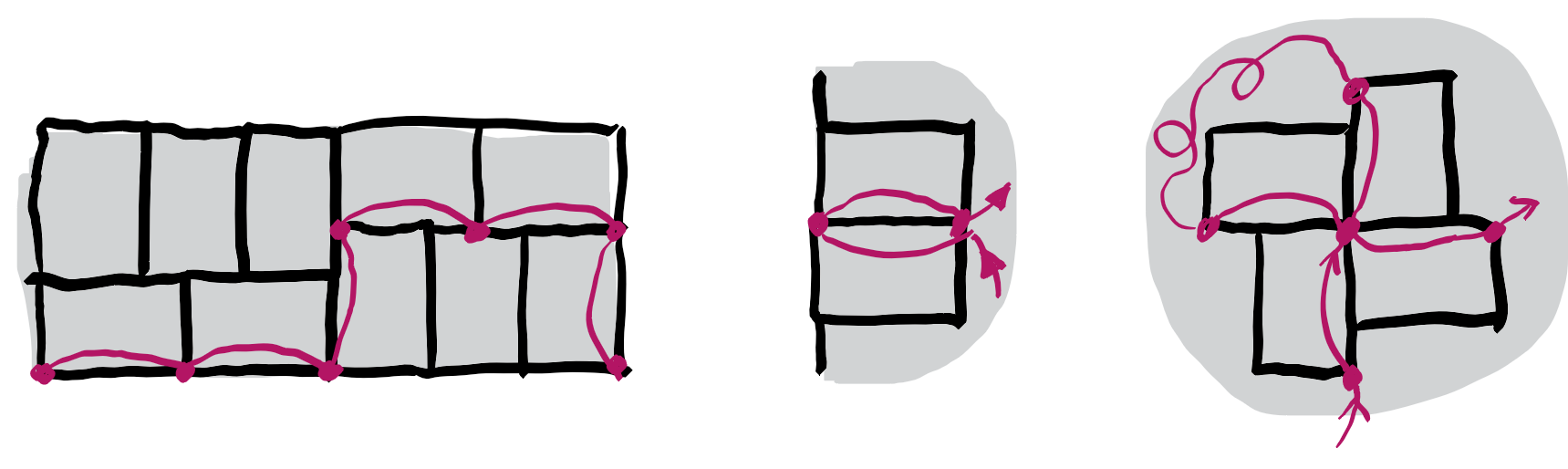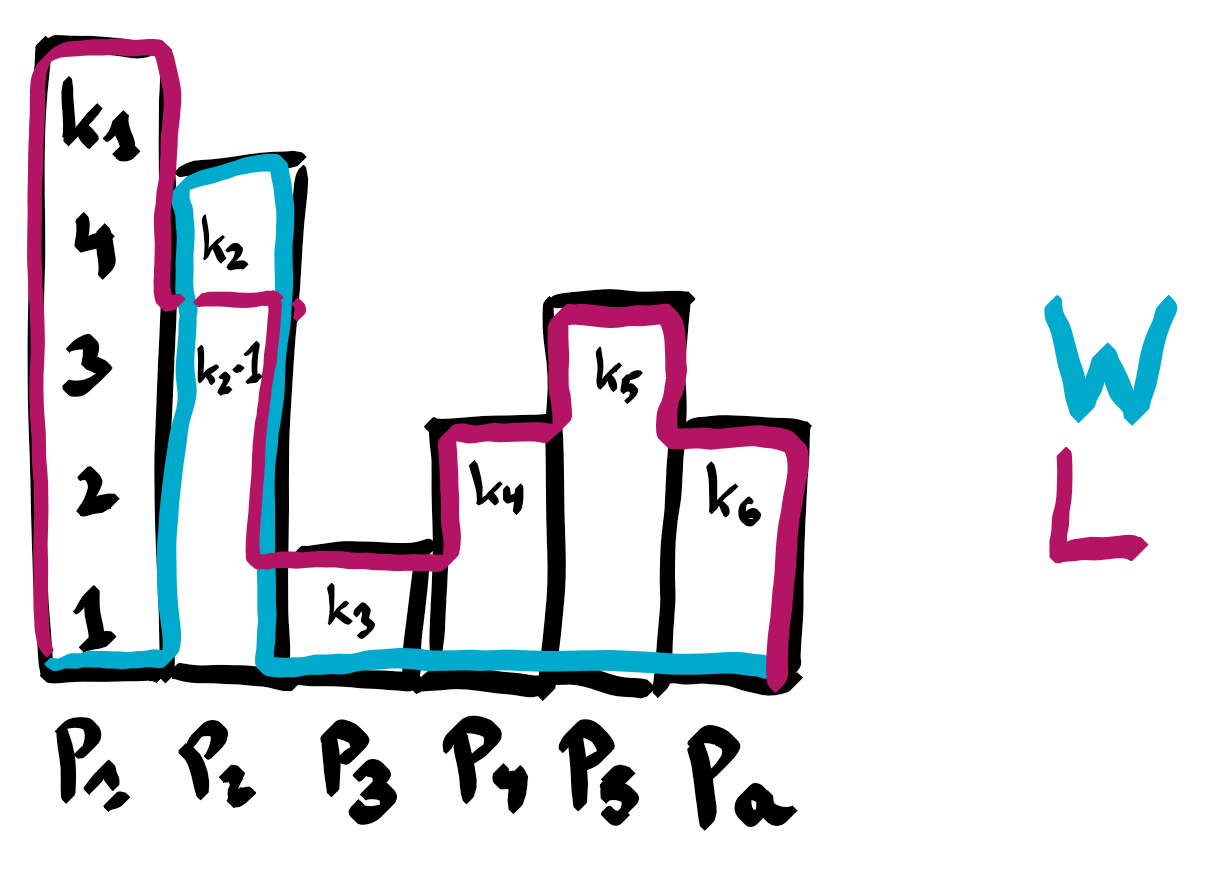A K by K grid has to be tiled with L by W tiles which can be taken vertically or horizontally. Given K find L, W such that this tiling is possible and (max(L,W)-min(L,W), L+W) is lexicographically maximal subject to W < L < K. All numbers are positive integers.

(this document has been updated in December 2021)

For every valid L, W pair we must have that L and W divide K. This is a theorem from the late 1960’s. At the end of this document is a link to 14 different proofs for this theorem, here is one. Given a fixed tiling of the grid, we construct a graph as follows. In fact it will be a multi-graph, as some edges might be present twice. The vertices consists of all points which are corners of individual tiles. For every tile, for each of its long side, there is an edge between the adjacent corners. This defines a graph with the following properties:

• the 4 corners of the grid have degree 1.
• all other points have degree 2 or 4.

Such a graph consists in a collection of paths and cycles. So if you start a walk from the lower left corner (with coordinates (0,0)), and make sure that you don’t walk over the same edge twice, then you must end in another corner of the grid. Such a corner has coordinates (0,K), (K,K) or (K,0). The coordinates of the intermediate points along the path all have coordinates which are multiplies of L. This proves that L divides K.The same argument can be used to show that W divides K as well. The problem statement required that no smaller square can be tiled, which means that K is the least common multiple (lcm for short) of W and L.

## Factorization of K

Suppose that K can be written as the product $p_1^{k_1}\cdots p_a^{k_a}$, for $a$ different primes, and positive integer exponents. Since both W and L divide K, they can be written as

$W = p_1^{w_1}\cdots p_a^{w_a}$

and

$L = p_1^{l_1}\cdots p_a^{l_a}.$Since lcm(W,L)=K, we have $\max\{w_i,l_i\}=k_i$ for every $i=1,\ldots,a$. Since L < K, we know that for at least one index $i$, we have $l_i < k_i$, which implies $w_i = k_i$. Since the difference L - W must be largest possible, we can assume $l_i = k_i-1$. Now we claim that for an optimal pair (L,W) we have $W = p_i^{k_i}$ and $L=K/p_i$. Indeed if for some index $j\neq i$, $l_j < k_j$, then $w_j=k_j$ and exchanging $l_j$ with $w_j$, increases the difference L - W, by the assumption W < L. Also if for some index $j\neq i$, $l_j=k_j$, then setting $w_j=0$ does not increase W.

## The algorithm

This means that we can solve the problem as follows.

• In a pre-processing step, generate all primes numbers up to a million, which is the square root of the upper bound on K.
• Decompose K into a product of prime factors $p_1^{k_1}\cdots p_a^{k_a}$.
• Return the pair $(L=K/p_i, W=p_i^{k_i})$ which maximizes L - W and in case of tie L + W.

## In Python

The implementation below does not pass the timelimits, which seem to be too harsh for Python. Here we implemented the sieve of Eratosthenes.

## In C++

Here we implemented the sieve of Gries-Misra, just for a change. But the sieve of Eratosthenes is good enough for this problem.

A related theorem with 14 proofs